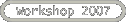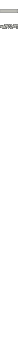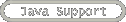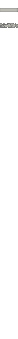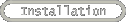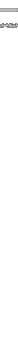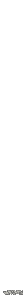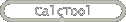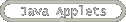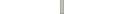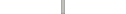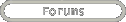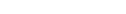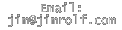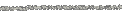If/then/else statements and switch statements are two primary ways to make decisions in Java.  We’ll talk primarily about if/then statements and leave you to refer to Appendix 5 for information needed to implement switch structures.

If/then/else statements
If/then statements have the general format

if (logical statement){
// put code statement(s) here
}
else{
//put more code statement(s) here
}

A logical statement is a statement that is either true or false.  Here are some examples:

• x<=y // is x less than or equal to y?
• x!=y // is x not equal to y?
• x!=y // is x not equal to y?
• x==y // is x equal to y?
• x||y // is x or y or both x and y true?
• x&&y // is x and y true?
• x>y   // is x strictly greater than y?
• x    // is x true? (This presumes x is a boolean variable)

For example, if we want to determine the maximum of two numbers, we might have code similar to the following.

double biggest=0.0;

if (x>y){//We decide here which one is the max.
biggest=x;
}
else{
biggest=y;
}

You are not required to utilize the else part of the if/then structure. So use it when it makes sense to you!

• Write code to examine the integer n inputted from nField and output an error message if n is negative.

See Sample Code 5 to check work.